6 minute read

# Relative References in Excel - A Beginner's GuideAgnes Lo

## What is a relative reference?

Relative reference Excel definition: A relative reference in Excel means there’s a point of reference applied to a cell or a formula where the return value is relative to the cell location.

This means the return value changes depending on where the cell or the formula moves to, within the same sheet or across different sheets. Relative reference is useful when the same calculation is required across different rows or columns.

Download your free practice file

Use this free Excel file to practice relative references along with the tutorial.

For example, to find out the total sales of each plant type sold, we would multiply Unit Price by Sold Qty (or =B2*C2) in Excel.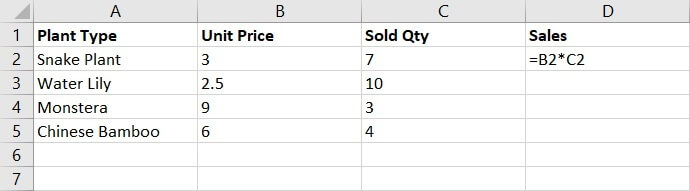When the cell references are being copied to the rest of the cells, they change simultaneously to multiply the Unit Price by Sold Quantity for each row, as shown in the image below.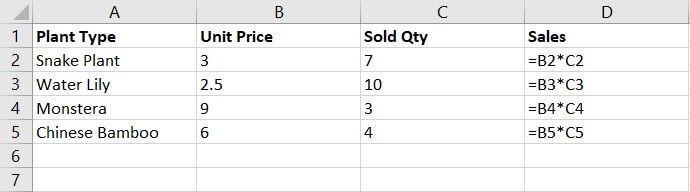In Excel, all references are relative by default. There’s no dollar sign (\$) required in the formula. Simply the relative row and column coordinates are required.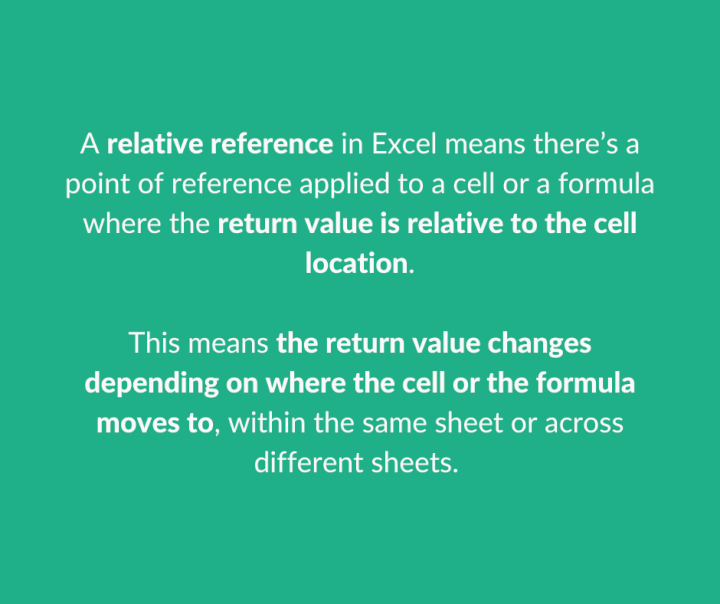## How to make a relative reference in Excel

Here are the steps on how to make a basic relative reference in Excel (=D3)

1. Choose a cell where you would like to create a relative reference. Cell D3 in this example: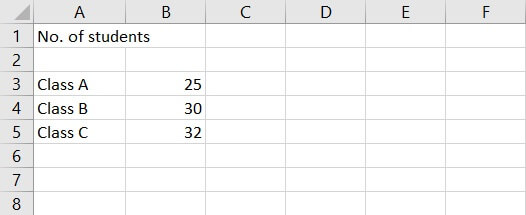1. In the formula of Cell D3, Enter “=” (the equal sign) and then select the point of reference — Cell B3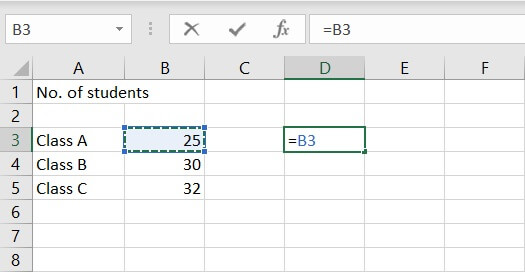Now Cell D3 is a relative reference to Cell B3. It will change accordingly if Cell B3 changes.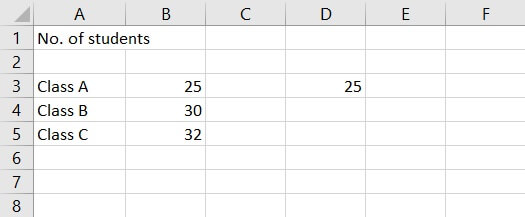## Examples of relative reference

Flexible in nature and easy to apply, relative reference is one of the most commonly used functions in Excel. It can be used with both numbers and text.

In particular, relative reference is frequently used when doing calculations in Excel, as it helps users to compute figures across scenarios and situations. It saves time by copying the blocks of cells across rows and columns too.

Below is an example of how to use relative reference:

A school health nurse has been asked to calculate students’ Body Mass Index. This is in order to assess the health risk of the students, whether they are in a healthy weight range. The weight (kg) and height (m) data of some students are already available.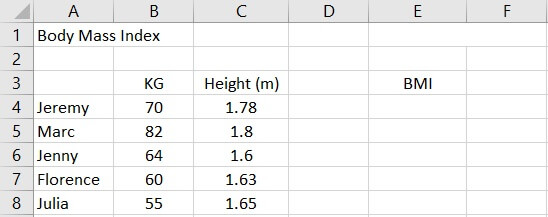To calculate the BMI, the formula is = kg/m2

In Jeremy’s case, it would be = 70/(1.78*1.78), or in Excel expression, it would be =B4/(C4*C4)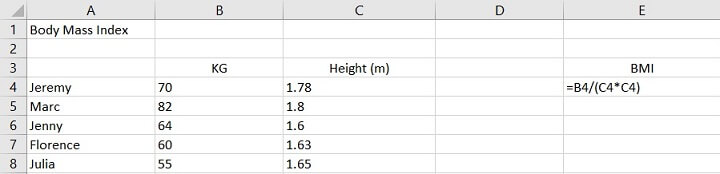The return value is 22.09317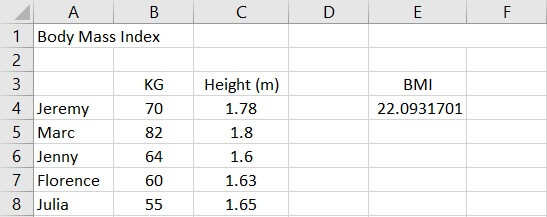To calculate the rest, simply copy and paste the formula in Cell E4 and apply to the rest of the cells (E5, E6, E7,E8).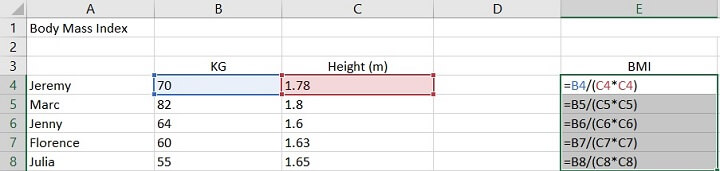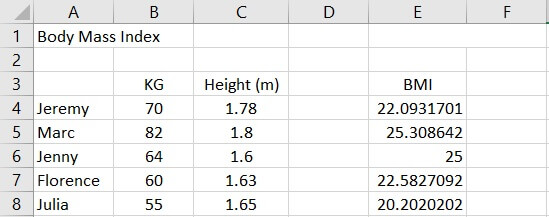In this example, the data sets are all variables. Each student comes in different weight and height. The only common ground is the calculation of their respective BMI, which requires us to apply the same formulas for each student.

Relative reference is particularly handy in such a situation where the constant is the calculation itself instead of the data set. It helps save time by copying the same formula across rows or columns, instead of manually inputting the formula for each scenario.

### Absolute reference vs relative reference in Excel

Let's take a look at the differences between relative and absolute references, with examples of when to use each.

#### Absolute reference Excel

A cell reference that is fixed in nature — a constant that does not change when being moved or copied across rows, columns or sheets.

For example:

At the end of a year, the CEO decides to give everyone the same amount of bonus payout (\$1,500). In this situation, \$1,500 is a fixed amount to add on top of everyone’s salary, regardless of their basic salary.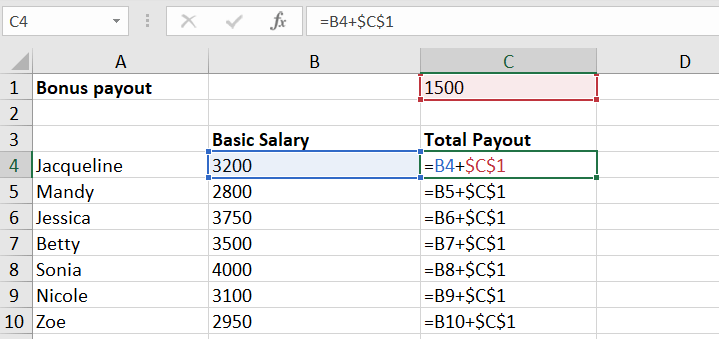For more examples of absolute references, check out this resource.

#### Relative reference Excel:

A cell reference that is always changing in nature — a variable that changes when being moved or copied across rows, columns or sheets.

For example:

Each type of food comes in with a different unit price and quantity. Their respective sales amount differs. Both unit price and quantity are variables.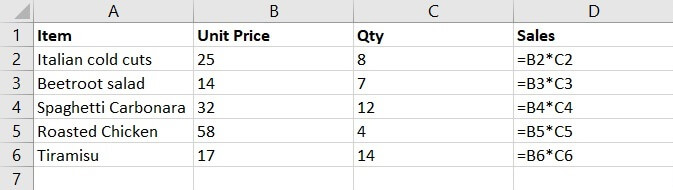## Summary

Relative reference in Excel refers to a constantly changing point of reference. The return value changes depending on where the cell or the formula moves to — within the same sheet or across different sheets. All cell references in Excel are relative references by default.

Unlike absolute reference, the dollar sign (\$) is absent in the formula, no cell is ‘locked’.

It is frequently used in Excel where repetitions of the same calculations or formulas are required for a range of data sets to compute scenarios and forecasts. It works with both numbers and text and helps to minimize errors.

To learn more about cell references and other useful Excel features, try our Excel - Basic and Advanced course now. Or start learning some Excel basics with our Excel in an Hour free course.

Learn Excel for free

Start learning formulas, functions, and time-saving hacks today with this free course!Agnes Lo

Agnes is a clienteling professional in luxury retail. She enjoys yoga and outdoor activities in her free time.

No comments